## Example 50.2 Computing Predicted Values for a Tobit Model

The LIFEREG procedure can be used to perform a Tobit analysis. The Tobit model, described by Tobin (1958), is a regression model for left-censored data assuming a normally distributed error term. The model parameters are estimated by maximum likelihood. PROC LIFEREG provides estimates of the parameters of the distribution of the uncensored data. See Greene (1993) and Maddala (1983) for a more complete discussion of censored normal data and related distributions. This example shows how you can use PROC LIFEREG and the DATA step to compute two of the three types of predicted values discussed there.

Consider a continuous random variable Y and a constant C. If you were to sample from the distribution of Y but discard values less than (greater than) C, the distribution of the remaining observations would be truncated on the left (right). If you were to sample from the distribution of Y and report values less than (greater than) C as C, the distribution of the sample would be left (right) censored.

The probability density function of the truncated random variable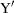is given by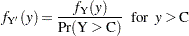where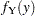is the probability density function of Y. PROC LIFEREG cannot compute the proper likelihood function to estimate parameters or predicted values for a truncated distribution. Suppose the model being fit is specified as follows: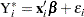where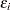is a normal error term with zero mean and standard deviation
.

Define the censored random variable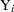as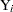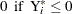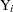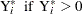This is the Tobit model for left-censored normal data.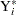is sometimes called the latent variable. PROC LIFEREG estimates parameters of the distribution ofby maximum likelihood.

You can use the LIFEREG procedure to compute predicted values based on the mean functions of the latent and observed variables. The mean of the latent variableis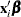, and you can compute values of the mean for different settings of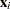by specifying XBETA=variable-name in an OUTPUT statement. Estimates offor each observation will be written to the OUT= data set. Predicted values of the observed variablecan be computed based on the mean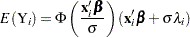where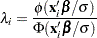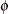and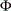represent the normal probability density and cumulative distribution functions.

Although the distribution ofin the Tobit model is often assumed normal, you can use other distributions for the Tobit model in the LIFEREG procedure by specifying a distribution with the DISTRIBUTION= option in the MODEL statement. One distribution that should be mentioned is the logistic distribution. For this distribution, the MLE has bounded influence function with respect to the response variable, but not the design variables. If you believe your data have outliers in the response direction, you might try this distribution for some robust estimation of the Tobit model.

With the logistic distribution, the predicted values of the observed variablecan be computed based on the mean of,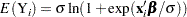The following table shows a subset of the Mroz (1987) data set. In these data, Hours is the number of hours the wife worked outside the household in a given year, Yrs_Ed is the years of education, and Yrs_Exp is the years of work experience. A Tobit model will be fit to the hours worked with years of education and experience as covariates.

Hours

Yrs_Ed

Yrs_Exp

0

8

9

0

8

12

0

9

10

0

10

15

0

11

4

0

11

6

1000

12

1

1960

12

29

0

13

3

2100

13

36

3686

14

11

1920

14

38

0

15

14

1728

16

3

1568

16

19

1316

17

7

0

17

15

If the wife was not employed (worked 0 hours), her hours worked will be left censored at zero. In order to accommodate left censoring in PROC LIFEREG, you need two variables to indicate censoring status of observations. You can think of these variables as lower and upper endpoints of interval censoring. If there is no censoring, set both variables to the observed value of Hours. To indicate left censoring, set the lower endpoint to missing and the upper endpoint to the censored value, zero in this case.

The following statements create a SAS data set with the variables Hours, Yrs_Ed, and Yrs_Exp from the preceding data. A new variable, Lower, is created such that Lower=. if Hours=0 and Lower=Hours if Hours>0.

```data subset;
input Hours Yrs_Ed Yrs_Exp @@;
if Hours eq 0
then Lower=.;
else Lower=Hours;
datalines;
0 8 9 0 8 12 0 9 10 0 10 15 0 11 4 0 11 6
1000 12 1 1960 12 29 0 13 3 2100 13 36
3686 14 11 1920 14 38 0 15 14 1728 16 3
1568 16 19 1316 17 7 0 17 15
;
run;
```

The following statements fit a normal regression model to the left-censored Hours data with Yrs_Ed and Yrs_Exp as covariates. You need the estimated standard deviation of the normal distribution to compute the predicted values of the censored distribution from the preceding formulas. The data set OUTEST contains the standard deviation estimate in a variable named _SCALE_. You also need estimates of. These are contained in the data set OUT as the variable Xbeta.

```proc lifereg data=subset outest=OUTEST(keep=_scale_);
model (lower, hours) = yrs_ed yrs_exp / d=normal;
output out=OUT xbeta=Xbeta;
run;
```

Output 50.2.1 shows the results of the model fit. These tables show parameter estimates for the uncensored, or latent variable, distribution.

Output 50.2.1 Parameter Estimates from PROC LIFEREG
The LIFEREG Procedure

Model Information
Data Set WORK.SUBSET
Dependent Variable Lower
Dependent Variable Hours
Number of Observations 17
Noncensored Values 8
Right Censored Values 0
Left Censored Values 9
Interval Censored Values 0
Number of Parameters 4
Name of Distribution Normal
Log Likelihood -74.9369977

Analysis of Maximum Likelihood Parameter Estimates
Parameter DF Estimate Standard Error 95% Confidence Limits Chi-Square Pr > ChiSq
Intercept 1 -5598.64 2850.248 -11185.0 -12.2553 3.86 0.0495
Yrs_Ed 1 373.1477 191.8872 -2.9442 749.2397 3.78 0.0518
Yrs_Exp 1 63.3371 38.3632 -11.8533 138.5276 2.73 0.0987
Scale 1 1582.870 442.6732 914.9433 2738.397

The following statements combine the two data sets created by PROC LIFEREG to compute predicted values for the censored distribution. The OUTEST= data set contains the estimate of the standard deviation from the uncensored distribution, and the OUT= data set contains estimates of.

```data predict;
drop lambda _scale_ _prob_;
set out;
if _n_ eq 1 then set outest;
lambda = pdf('NORMAL',Xbeta/_scale_)
/ cdf('NORMAL',Xbeta/_scale_);
Predict = cdf('NORMAL', Xbeta/_scale_)
* (Xbeta + _scale_*lambda);
label Xbeta='MEAN OF UNCENSORED VARIABLE'
Predict = 'MEAN OF CENSORED VARIABLE';
run;
```

Output 50.2.2 shows the original variables, the predicted means of the uncensored distribution, and the predicted means of the censored distribution.

Output 50.2.2 Predicted Means from PROC LIFEREG
Hours Lower Yrs_Ed Yrs_Exp MEAN OF UNCENSORED
VARIABLE
MEAN OF CENSORED
VARIABLE
0 . 8 9 -2043.42 73.46
0 . 8 12 -1853.41 94.23
0 . 9 10 -1606.94 128.10
0 . 10 15 -917.10 276.04
0 . 11 4 -1240.67 195.76
0 . 11 6 -1113.99 224.72
1000 1000 12 1 -1057.53 238.63
1960 1960 12 29 715.91 1052.94
0 . 13 3 -557.71 391.42
2100 2100 13 36 1532.42 1672.50
3686 3686 14 11 322.14 805.58
1920 1920 14 38 2032.24 2106.81
0 . 15 14 885.30 1170.39
1728 1728 16 3 561.74 951.69
1568 1568 16 19 1575.13 1708.24
1316 1316 17 7 1188.23 1395.61
0 . 17 15 1694.93 1809.97